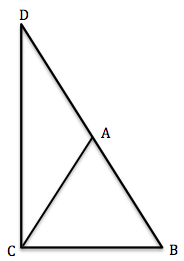### ABC is an isosceles triangle with AB = AC. We extend the segment BA to D such that BA = AD. What is the value of ∠BCD?

90°

Step by Step Explanation:
1. Take a look at the image below:ABC is an isosceles triangle with AB = AC,
therefore, ∠ABC = ∠ACB = x
Let ∠CAB = y. Then y + x + x = 180°
⇒ y + 2x = 180°
⇒ ∠ACB = x =
 180°-y 2

2. Since we extended BA to form line BD, ∠BAC + ∠DAC = 180°
⇒ y + ∠DAC = 180°
⇒ ∠DAC = 180° - y
3. CAD is also an isosceles triangle since AD = AC (remember AD = AB and AB = AC)
So ∠CDA = ∠DCA = z
⇒ ∠CDA + ∠DCA + ∠DAC = 180°
⇒ z + z + (180°-y) = 180°
⇒ 2z = y
⇒ ∠DCA = z =
 y 2

4. Now, ∠DCB = ∠DCA + ∠ACB =
 180°-y 2
+
 y 2
= 90°